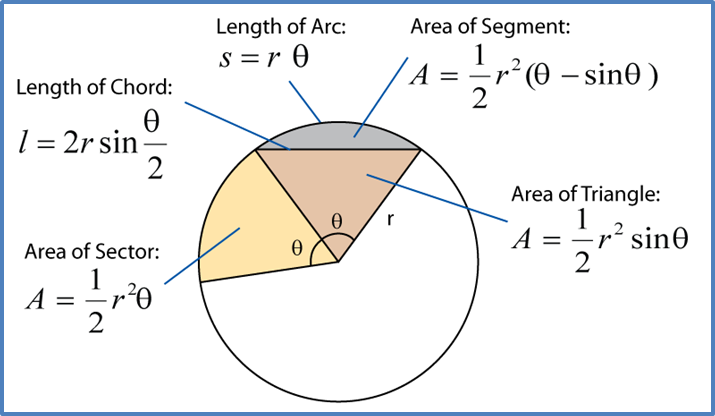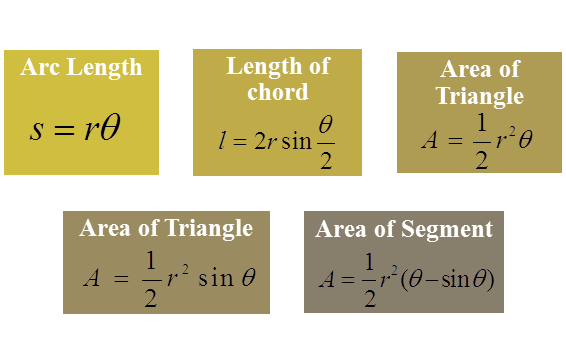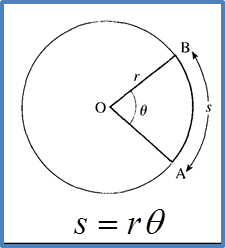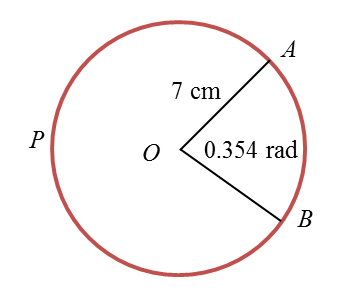# 8.2 Length of an Arc of a Circle

(A) Formulae for Length and Area of a Circler = radius, A= area, s = arc length, q = angle, l = length of chord(B) Length of an Arc of a CircleExample 1:
An arc, AB, of a circle of radius 5 cm subtends an angle of 1.5 radians at the centre.  Find the length of the arc AB.

Solution:
s = rθ
Length of the arc AB = (5)(1.5) = 7.5 cm

Example 2:
An arc, PQ, of a circle of radius 12 cm subtends an angle of 30° at the centre.  Find the length of the arc PQ.

Solution:
Length of the arc PQ

Example 3:In the above diagram, find
(i) length of the minor arc AB
(ii) length of the major arc APB

Solution:
(i) length of the minor arc AB = rθ
= (7)(0.354)
= 2.478 cm

(ii) Since 360o = 2π radians, the reflex angle AOB
1.